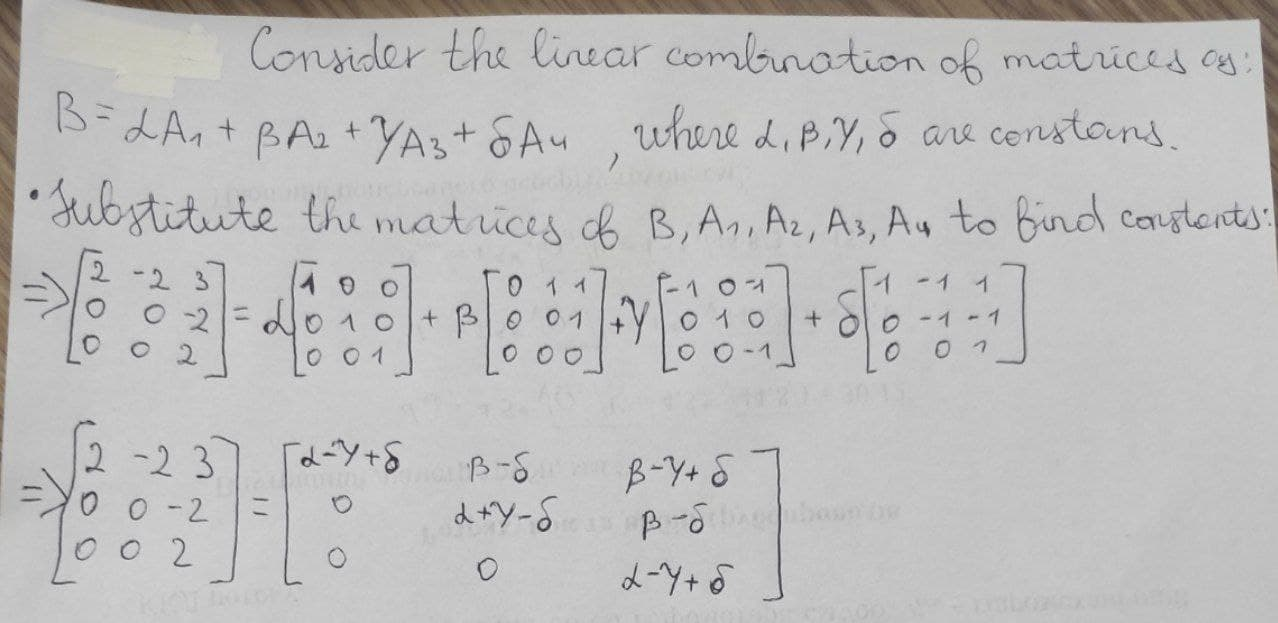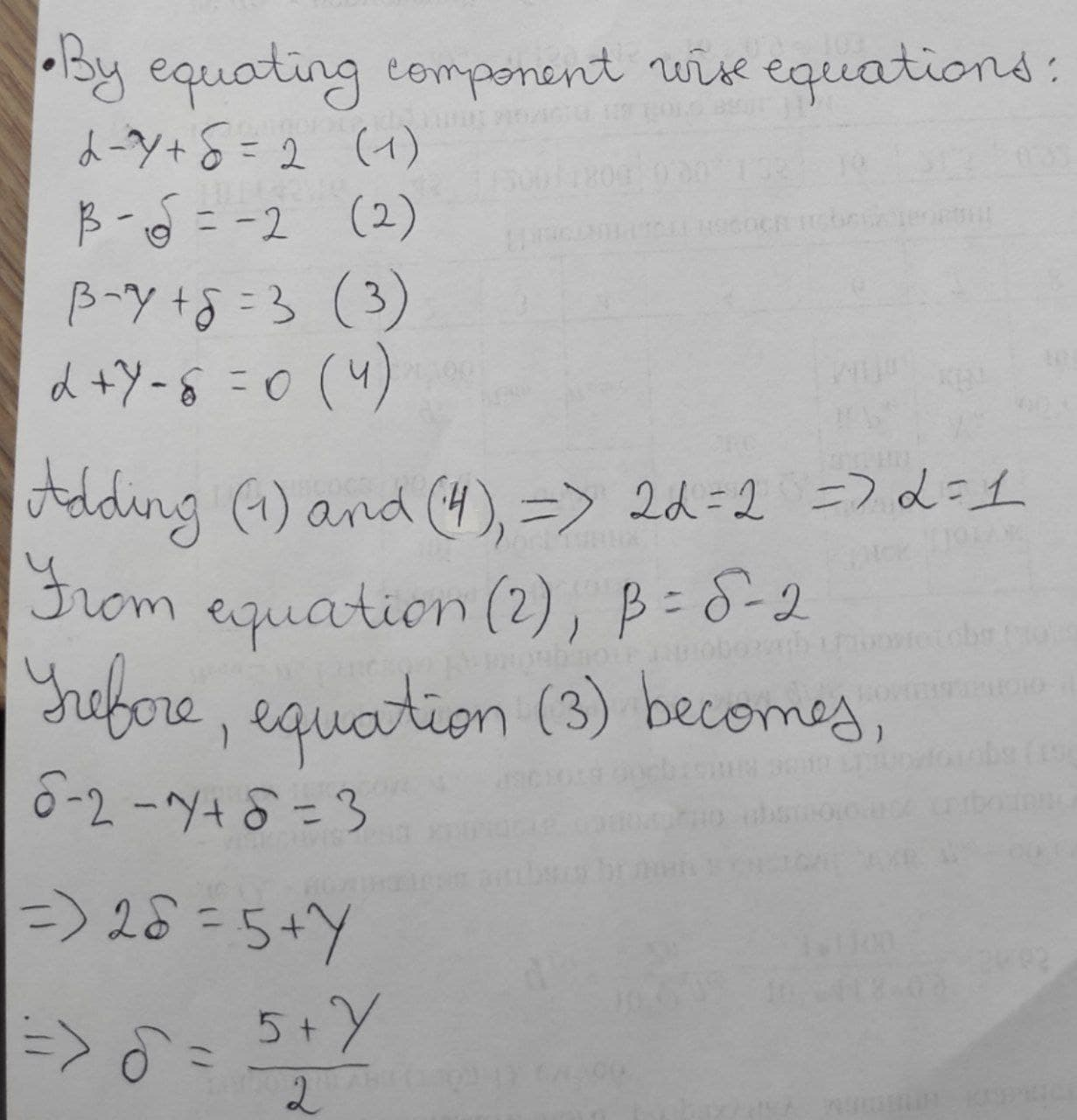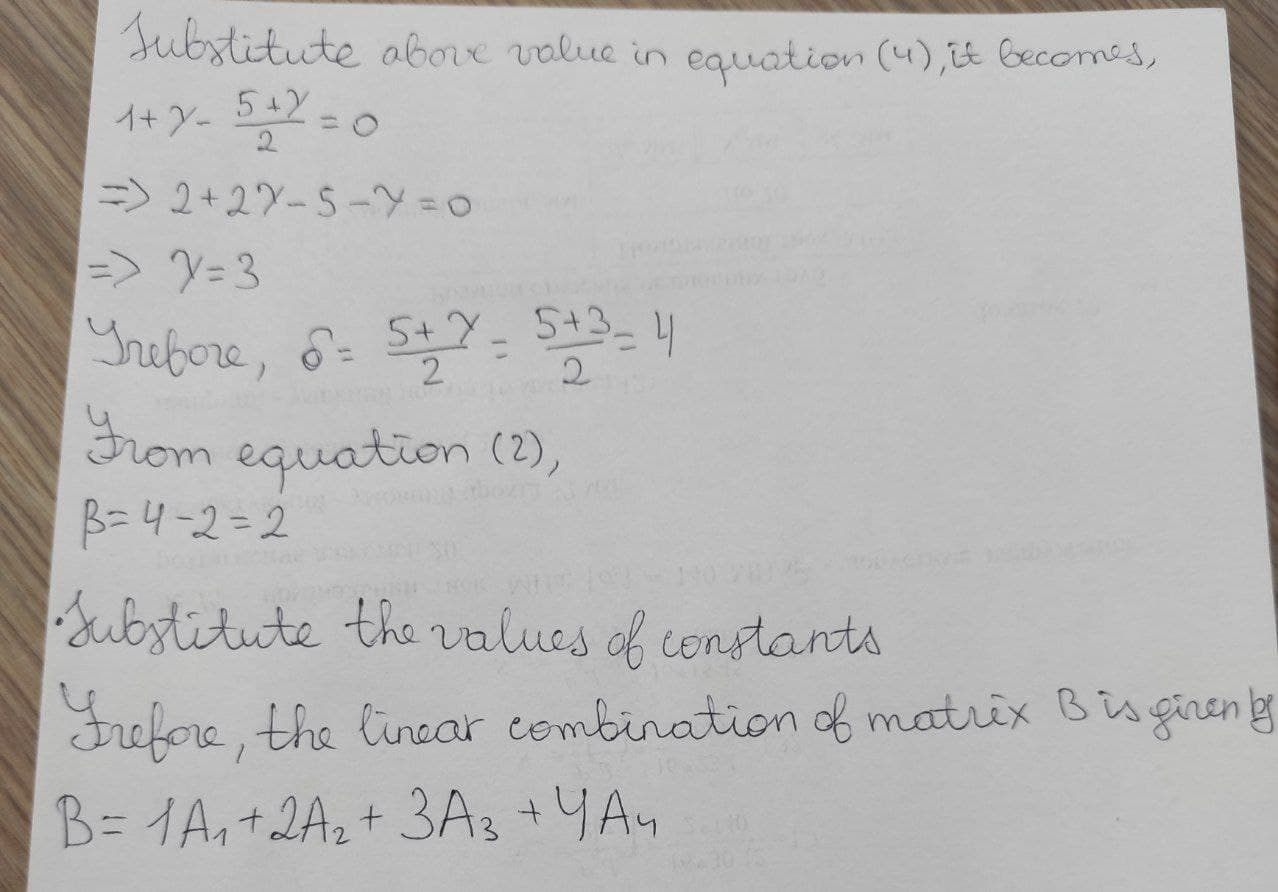Question# write B as a linear combination of the other matrices, if possible. B=[[2,-2,3],[0,0,-2],[0,0,2]] A_1=[[1,0,0],[0,1,0],[0,0,1]] A_2=[[0,1,1],[0,0,1],[0,0,0]] A_3=[[-1,0,-1],[0,1,0],[0,0,-1]] A_4=[[1,-1,1],[0,-1,-1],[0,0,1]]

Matrices
ANSWEREDwrite B as a linear combination of the other matrices, if possible.
$$B=[[2,-2,3],[0,0,-2],[0,0,2]]$$
$$A_1=[[1,0,0],[0,1,0],[0,0,1]]$$
$$A_2=[[0,1,1],[0,0,1],[0,0,0]]$$
$$A_3=[[-1,0,-1],[0,1,0],[0,0,-1]]$$
$$A_4=[[1,-1,1],[0,-1,-1],[0,0,1]]$$